Download Presentation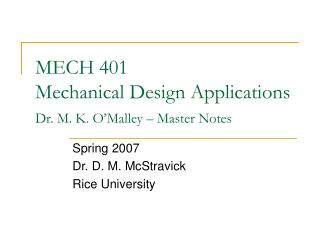MECH 401 Mechanical Design Applications Dr. M. K. O’Malley – Master Notes

# MECH 401 Mechanical Design Applications Dr. M. K. O’Malley – Master Notes - PowerPoint PPT Presentation

MECH 401 Mechanical Design Applications Dr. M. K. O’Malley – Master Notes. Spring 2007 Dr. D. M. McStravick Rice University. Design Considerations. Stress – Yield Failure or Code Compliance Deflection Strain Stiffness Stability – Important in compressive membersI am the owner, or an agent authorized to act on behalf of the owner, of the copyrighted work described.
Download Presentation## MECH 401 Mechanical Design Applications Dr. M. K. O’Malley – Master Notes

An Image/Link below is provided (as is) to download presentation

Download Policy: Content on the Website is provided to you AS IS for your information and personal use and may not be sold / licensed / shared on other websites without getting consent from its author.While downloading, if for some reason you are not able to download a presentation, the publisher may have deleted the file from their server.

- - - - - - - - - - - - - - - - - - - - - - - - - - E N D - - - - - - - - - - - - - - - - - - - - - - - - - -
Presentation Transcript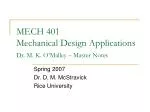### MECH 401 Mechanical Design ApplicationsDr. M. K. O’Malley – Master Notes

Spring 2007

Dr. D. M. McStravick

Rice University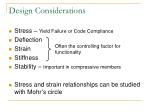Design Considerations
• Stress – Yield Failure or Code Compliance
• Deflection
• Strain
• Stiffness
• Stability – Important in compressive members
• Stress and strain relationships can be studied with Mohr’s circle

Often the controlling factor for functionality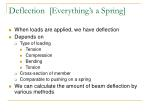Deflection [Everything’s a Spring]
• When loads are applied, we have deflection
• Depends on
• Tension
• Compression
• Bending
• Torsion
• Cross-section of member
• Comparable to pushing on a spring
• We can calculate the amount of beam deflection by various methods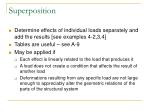Superposition
• Determine effects of individual loads separately and add the results [see examples 4-2,3,4]
• Tables are useful – see A-9
• May be applied if
• Each effect is linearly related to the load that produces it
• A load does not create a condition that affects the result of another load
• Deformations resulting from any specific load are not large enough to appreciably alter the geometric relations of the parts of the structural system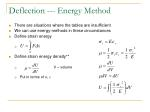Deflection --- Energy Method
• There are situations where the tables are insufficient
• We can use energy-methods in these circumstances
• Define strain energy
• Define strain energy density**
• V – volume
• Put in terms of s, e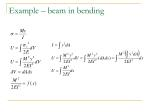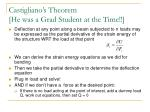Castigliano’s Theorem[He was a Grad Student at the Time!!]
• Deflection at any point along a beam subjected to n loads may be expressed as the partial derivative of the strain energy of the structure WRT the load at that point
• We can derive the strain energy equations as we did for bending
• Then we take the partial derivative to determine the deflection equation
• Plug in load and solve!
• AND if we don’t have a force at the desired point:
• If there is no load acting at the point of interest, add a dummy load Q, work out equations, then set Q = 0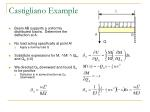Castigliano Example
• Beam AB supports a uniformly distributed load w. Determine the deflection at A.
• No load acting specifically at point A!
• Apply a dummy load Q
• Substitute expressions for M, M/ QA, and QA (=0)
• We directed QA downward and foundδA to be positive
• Defection is in same direction as QA (downward)

Q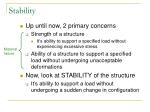Stability
• Up until now, 2 primary concerns
• Strength of a structure
• It’s ability to support a specified load without experiencing excessive stress
• Ability of a structure to support a specified load without undergoing unacceptable deformations
• Now, look at STABILITY of the structure
• It’s ability to support a load without undergoing a sudden change in configuration

Material

failure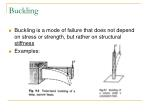Buckling
• Buckling is a mode of failure that does not depend on stress or strength, but rather on structural stiffness
• Examples:Buckling
• The most common problem involving buckling is the design of columns
• Compression members
• The analysis of an element in buckling involves establishing a differential equation(s) for beam deformation and finding the solution to the ODE, then determining which solutions are stable
• Euler solved this problem for columnsEuler Column Formula
• Where C is as follows:

C = ¼ ;Le=2L

Fixed-free

C = 2; Le=0.7071L

Fixed-pinned

C = 1: Le=L

Rounded-rounded

Pinned-pinned

C = 4; Le=L/2

Fixed-fixedBuckling
• Geometry is crucial to correct analysis
• Euler – “long” columns
• Johnson – “intermediate” length columns
• Determine difference by slenderness ratio
• The point is that a designer must be alert to the possibility of buckling
• A structure must not only be strong enough, but must also be sufficiently rigidSolving buckling problems
• Find Euler-Johnson tangent point with
• For Le/r < tangent point (“intermediate”), use Johnson’s Equation:
• For Le/r > tangent point (“long”), use Euler’s equation:
• For Le/r < 10 (“short”), Scr =Sy
• If length is unknown, predict whether it is “long” or “intermediate”, use the appropriate equation, then check using the Euler-Johnson tangent point once you have a numerical solution for the critical strengthSpecial Buckling Cases
• Buckling in very long Pipe
• Note Pcrit is inversely related to length squared
• A tiny load will cause buckling
• L = 10 feet vs. L = 1000 feet:
• Pcrit1000/Pcrit10 = 0.0001
• Buckling under hydrostatic PressureImpact
• Impact – Chapter 4
• Fatigue – Chapter 6
• Examples?
• 3 categories
• Rapidly moving loads of constant magnitude
• Driving over a bridge
• Suddenly applied loads
• Explosion, combustion
• Direct impact
• Pile driver, jack hammer, auto crash

Increasing

SeverityImpact, cont.
• It is difficult to define the time rates of load application
• Leads to use of empirically determined stress impact factors
• If t is time constant of the system, where
• We can define the load type by the time required to apply the load (tAL = time required to apply the load)
• Static
• “Gray area”
• DynamicStress and deflection due to impact
• W – freely falling mass
• k – structure with stiffness (usually large)
• Assumptions
• Mass of structure is negligible
• Deflections within the mass are negligible
• Damping is negligible
• Equations are only a GUIDE
• h is height of freely falling mass before its release
• d is the amount of deflection of the spring/structureEnergy balance
• Fe is the equivalent static force necessary to create an amount of deflection equal to d
• Energy Balance of falling weight, WImpact, cont.
• Sometimes we know velocity at impact rather than the height of the fall
• An energy balance gives: